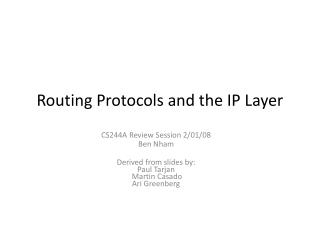Download PresentationRouting Protocols and the IP LayerRouting Protocols and the IP Layer - PowerPoint PPT Presentation

Download PresentationRouting Protocols and the IP Layer
An Image/Link below is provided (as is) to download presentation

Download Policy: Content on the Website is provided to you AS IS for your information and personal use and may not be sold / licensed / shared on other websites without getting consent from its author. While downloading, if for some reason you are not able to download a presentation, the publisher may have deleted the file from their server.

- - - - - - - - - - - - - - - - - - - - - - - - - - - E N D - - - - - - - - - - - - - - - - - - - - - - - - - - -
Presentation Transcript

1. Routing Protocols and the IP Layer CS244A Review Session 2/01/08 Ben Nham Derived from slides by: Paul Tarjan Martin CasadoAri Greenberg

2. Functions of a router • Forwarding • Determine the correct egress port for an incoming packet based on a forwarding table • Routing • Choosing the best path to take from source to destination • Routing algorithms build up forwarding tables in each router • Two main algorithms: Bellman-Ford and Dijkstra’s Algorithm

3. Bellman Ford Equation • Let G = (V, E) and Cost(e) = cost of edge e • Suppose we want to find the lowest cost path to vertex t from all other vertices in V • Let Shortest-Path(i, u) be the shortest path from u to t using at most iedges. Then: • If there are no negative edge costs, then any shortest path has at most |V|-1 edges. Therefore, algorithm terminates after |V|-1 iterations.

4. Bellman Ford Algorithm function BellmanFord(list vertices, list edges, vertex dest) // Step 1: Initialize shortest paths of w/ at most 0 edges for each vertex v in vertices: v.next := null if v is dest: v.distance := 0 else: v.distance := infinity // Step 2: Calculate shortest paths with at most i edges from // shortest paths with at most i-1 edges for i from 1 to size(vertices) - 1: for each edge (u,v) in edges: if u.distance > Cost(u,v) + v.distance: u.distance = Cost(u,v) + v.distance u.next = v

5. An Example A B C 2 1 3 1 9 1 2 D E F

6. Example A B C 2 1 9 5 1 1 2 D E F

7. Solution A B C 2 1 1 1 2 D E F

8. Bellman-Ford and Distance Vector • We’ve just run a centralized version of Bellman-Ford • Can be distributed as well, as described in lecture and text • In distributed version: • Maintain a distance vector that maintains cost to all other nodes • Maintain cost to each directly attached neighbor • If we get a new distance vector or cost to a neighbor, re-calculate distance vector, and broadcast new distance vector to neighbors if it has changed • For any given router, who does it have to talk to? • What does runtime depend on?

9. Problems with Distance Vector A B C • Increase in link cost is propagated slowly • Can “count to infinity” • What happens if we delete (B, C)? • B now tries to get to C through A, and increase its cost to C • A will see that B’s cost of getting to C increased, and will increase its cost • Shortest path to C from B and A will keep increasing to infinity • Partial solutions • Set infinity • Split horizon • Split horizon with poison reverse 2 1

10. Dijkstra’s Algorithm • Given a graph G and a starting vertex s, find shortest path from s to any other vertex in G • Use greedy algorithm: • Maintain a set S of nodes for which we know the shortest path • On each iteration, grow S by one vertex, choosing shortest path through S to any other node not in S • If the cost from S to any other node has decreased, update it

11. Dijkstra’s Algorithm function Dijkstra(G, w, s) Q = new Q // Initialize a priority queue Q for each vertex v in V[G] // Add every vertex to Q with inf. cost d[v] := infinity previous[v] := undefined Insert(Q, v, d[v]) d[s] := 0 // Distance from s to s ChangeKey(Q, s, d[s]) // Change value of s in priority queue S := empty set // Set of all visited vertices while Q is not an empty set // Remove min vertex from priority queue, mark as visited u := ExtractMin(Q) S := S union {u} // Relax (u,v) for each edge for each edge (u,v) outgoing from u if d[u] + w(u,v) < d[v] d[v] := d[u] + w(u,v) previous[v] := u ChangeKey(Q, v, d[v])

12. Example A B C 2 1 9 3 1 1 2 D E F

13. Solution A B C 2 1 9 3 1 1 2 D E F

14. Link-State (Using Dijkstra’s) • Algorithm must know the cost of every link in the network • Each node broadcasts LS packets to all other nodes • Contains source node id, costs to all neighbor nodes, TTL, sequence # • If a link cost changes, must rebroadcast • Calculation for entire network is done locally

15. Comparison between LS and DV • Messages • In link state: Each node broadcasts a link state advertisement to the whole network • In distance vector: Each node shares a distance vector (distance to every node in network) to its neighbor • How long does it take to converge? • O((|E|+|V|) log |V|) = O(|E| log |V|) for Dijkstra’s • O(|E||V|) for centralized Bellman-Ford; for distributed, can vary • Robustness • An incorrect distance vector can propagate through the whole network